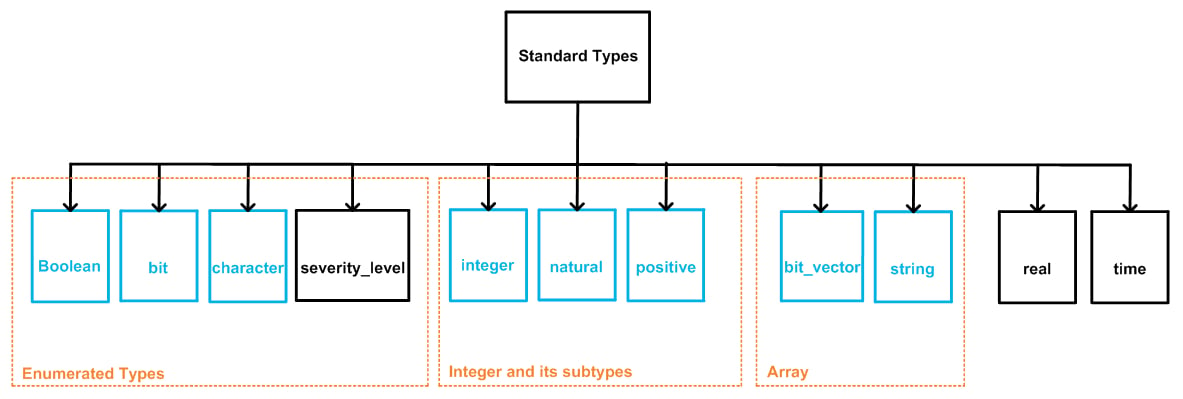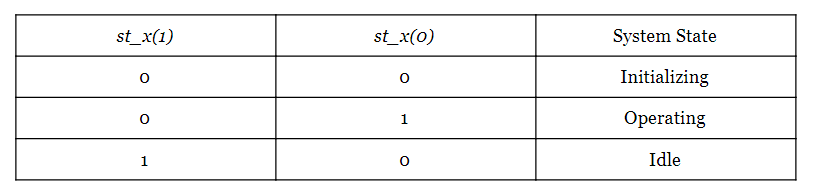Technical Article

# VHDL Data Types: Some Classifications and the Enumerated Type

January 24, 2018 by Dr. Steve Arar

## This article will first review classifications for the VHDL data types, and then it will discuss the enumerated type.

This article will first review classifications for the VHDL data types, and then it will discuss the enumerated type.

VHDL provides us with several options for the data type of objects. In previous articles, we looked at the std_logic and std_logic_vector types. This article will first review classifications for the VHDL data types, and then it will discuss the enumerated type.

### Classifying VHDL Data Types

Classifying VHDL data types helps to develop a better understanding of the topic. A common classification method is shown in Figure 1.##### Figure 1. Each VHDL data-type group (shown in orange) is defined in a VHDL package (shown in blue).

In this figure, data type classification is based on the package that defines the type. VHDL packages are shown in blue in the figure. Each of these packages includes the definition for some VHDL data types (which are shown in orange).

For example, the “std_logic” and “std_logic_vector” data types are “standard_logic types”. As shown in Figure 1, these types originate from the “std_logic_1164” package. We saw previously that to use the “std_logic” and “std_logic_vector” data types, we need to include the “std_logic_1164” package from the “ieee” library in our code. That is, the following lines of code are required.

1	library ieee;
2	use ieee.std_logic_1164.all

Similarly, before using any other data type, we need to make the corresponding package visible to the code. This article will review the enumerated data types. There are several predefined enumerated types in the “standard types” category from the “standard” package. This package is included in the “std” VHDL library. So, to use the “standard types”, we must make the “std” library and the “standard” package visible to the design:

1	library std;
2	use std.standard.all

However, since the above library and package are frequently used, they are added to the code by default. Hence, there is no need to explicitly include the above statements in our code. Now, let’s look at the “standard types” section of Figure 1 in more detail.

### The Standard Types

There are several data types in this category. These are shown in Figure 2 below.##### Figure 2. Different data types in the category “standard types”.

As shown in this figure, some of these types can be grouped together. For example, the types “boolean”, “bit”, “character”, and “severity_level” can be grouped as “enumerated types” and so on. This article will explain and provide examples for the enumeration data type, and in future articles we will continue to discuss the data types shown in Figure 2.

### Enumerated Types

The enumerated data types can be best explained by means of an example. Assume that you’re designing a digital system that can be in one of the following three states: initializing, operating, and idle. In each of these states the system performs certain operations. For example, in the initializing state, the system may update its inputs. In the operating state, it may perform some arithmetic operations. In the idle state, it may go to a low-power mode. We don’t care what condition makes the system go from a particular state to another one. What matters to us is how to represent the state of the system. One way can be representing the state of the system using a two-bit signal, e.g., st_x. The signal st_x can be defined as a two-element “std_logic_vector” data type in VHDL. We may consider each state of this signal as a code for the state of the system; for example:We can check st_x continuously and perform the required operations based on the state of the system which is coded into the value of st_x. For example, assume that, in the operating state, the system is supposed to AND two inputs a1 and a2 and assign the result to the output out1. However, in the other two states, out1 is supposed to be equal to a third input called a3. The following code can be used to describe the behavior of the output out1 (for more details about concurrent assignments see this article).

1	with st_x select
2		out1 <= (a1 and a2) when "01",
3			 a3 when others;

The problem with this method is that we need to remember what code is used to represent each state of the system. This increases the chance of error. What if we could use words instead of a string of ones and zeros to represent the states of the system in our code? Words have much more meaning for us humans than zeros and ones. This is, in fact, what the VHDL enumeration data type can do.

The enumerated data type can be used to define a signal that has words as its values. For example, we can define st_x as a signal that can take on three values: “st_initializing”, “st_operating”, and “st_idle”. (You could use different words, but of course you want to choose identifiers that make the code more clear and intuitive.) However, we need to first define the data type itself before we define a signal of this type. A logical choice for the name of this data type would be “sys_state”. Signals of this data type can take on three values: “st_initializing”, “st_operating”, and “st_idle”. The following code can be used to define this new data type.

1	type sys_state is (st_initializing, st_operating, st_idle);

As you can see, the data type name, “sys_state”, comes between the keywords “type” and “is”. The values defined for this data type are enclosed in parentheses. This is called an enumeration data type, because the values are enumerated on a list. Now, we can define a signal, st_x, of the type “sys_state”.

2	signal st_x : sys_state;

Note that st_x can take on only the values defined for the “sys_state” type. Now that we have this enumerated data type, the following code can be used to describe the behaviour of the output out1 in the above example.

1	with st_x select
2		out1 <= (a1 and a2) when st_operating,
3		        a3 when others;

Figure 3 shows a Xilinx ISE simulation for the above “with/select” assignment. As you can see, from 200 ns to 400 ns, the signal st_x is equal to “st_operating” and, hence, out1 is equal to a1 AND a2. For other values of st_x, the output follows the value of a3.##### Figure 3.

Similar to what we do with other data types, we can use the assignment operator to assign values to the st_x signal. For example, to assign the value “st_initializing” to st_x, we can write:

st_x <= st_initializing;

### Several Enumerated Types Having a Common Value

It is possible to have common values between two different user-defined enumerated data types. For example, assume that you define the following enumerated data types to describe the state of two subsystems in your design:

1	type sys_stateA is (st_initializing, st_operating, st_idle);
2	type sys_stateB is (st_multiply, st_add, st_idle);
3	signal st_xA : sys_stateA;
4	signal st_xB : sys_stateB;

As you can see, the literal value “st_idle” is common between the two types (“literal” refers to a value that is inserted by the designer directly into the VHDL code). However, VHDL considers this acceptable because we are dealing with two different data types, namely, “sys_stateA” and “sys_stateB”. If we assign "st_idle" to the signals st_xA and st_xB, the utilized data type makes it clear which "st_idle" is meant. Hence, both of the following assignments will be valid:

5	st_xA <= st_idle;
6	st_xB <= st_idle;

### The Initial Value of Enumerated Types

The leftmost element of the enumeration list will be considered as the default value, i.e., the initial value that is assigned to objects of that type. For example, the default value of st_xA and st_xB will be "st_initializing" and "st_multiply", respectively.

### Enumeration of Character Literals

Enumeration types can also be defined using character literals. In this case, we must enclose each character in single quotes. For example, the following code describes a type which has three character literals.

1	type char_enum is (‘c’, ‘z’, ‘1’);

As shown in Figure 2, the types “boolean”, “bit”, and “character” are predefined enumerated types. The type “bit” was briefly discussed in a previous article. This type can take on either the character ‘0’ or ‘1’. The type definitions for the predefined data types "bit" and "boolean" are given below.

type bit is (‘0’, ‘1’);
type boolean is (false, true);

### Summary

• One common way of classifying the VHDL data types is a classification based on the package that defines the type.
• Since the “std” library and the “standard” package are frequently used, they are added to the code by default. Hence, there is no need to explicitly make these visible to the design.
• The types “boolean”, “bit”, “character”, and “severity_level” are the enumerated types available in the “standard” package.
• Instead of using bit-level encoding, we can use enumerated data types to describe the design on a more abstract level.
• It is possible to have common values between two different user-defined enumerated data types.
• The leftmost element of the enumeration list is the default value assigned to objects of an enumerated data type.